Search    VB VB.net C# ASP.net ASP SQL Server VC++ My Links Networking ORACLE MySQL PHP Unix/Linux WinNT/2k/2003 Flash Maya 3D max Photoshop DB2 C/C++/ASM Microcontroller Circuit Design JAVA Wireless/Mobile JScript     Articles from this site only Links only Articles with downloads Articles with screenshot(s)  Code/Page 10 20 30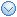User Login Email Password Remember Me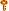Forgot the Password?Services
» Web Development
» Maintenance
» Data Integration/BI
» Information ManagementProgrammingDatabaseAutomationOS/NetworkingGraphicsLinksTools
» Regular Expr Tester
» Free Tools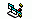Working with LZ (Lempel-Ziv) compression APIs

Total Hit ( 5019)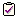Rate this article:     Poor     ExcellentSubmit Your Question/Comment about this article

RatingClick here to download the attached file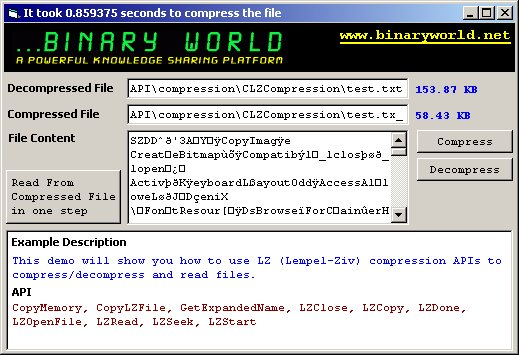Lempel-Ziv compression is a lossless compression algorithm, which means that no data is lost when compressing and decompressing the file, as opposed to lossy compression algorithms such as JPEG, where some data is lost each time data compression and decompression occur. NTFS volumes support file compression on an individual file basis. The file compression algorithm used by NTFS is Lempel-Ziv compression. Each type of data-compression algorithm minimizes redundant data in a unique manner. For example, the Huffman encoding algorithm assigns a code to characters in a file based on how frequently those characters occur. Another compression algorithm, called run-length encoding, generates a two-part value for repeated characters: the first part specifies the number of times the character is repeated, and the second part identifies the character. Another compression algorithm, known as the Lempel-Ziv algorithm, converts variable-length strings into fixed-length codes that consume less space than the original strings.In this article I will show you how to create a very simple to use class which you can use to compress and decompress files using LZ Compression method.Step-By-Step Example- Create a standard exe project- Add one class module and rename it to CLZCompression- Add 3 commandbutton controls on the form1- Add 3 textbox controls on the form1 (for text3 set MultiLine=True and scroolbar=verticle)- Add two label controls- Add the following code in form1Form1.frm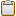Click here to copy the following block '//This sample will work 8 times faster if you run compiled versionDim lzComp As New CLZCompressionPrivate Sub Command1_Click()  Dim t  t = Timer  Me.Caption = "Compressing the file..."  If lzComp.Compress(Text1, Text2) = True Then    Me.Caption = "It took " & Timer - t & " seconds to compress the file"    MsgBox "Compressed file path : " & Text2, vbInformation    Label1.Caption = Round(FileLen(Text1) / 1000, 2) & " KB"    Label2.Caption = Round(FileLen(Text2) / 1000, 2) & " KB"    '//Textbox only look up to first null character so whe have to remove all nulls to display full content    Text3 = Replace(FileText(Text2), vbNullChar, "")  '//Compressed file content (Which wont be readable)  Else    MsgBox "Error in compress file", vbCritical    Me.Caption = "Failed to compress"  End IfEnd SubPrivate Sub Command2_Click()  Dim t  t = Timer  Me.Caption = "Decompressing the file..."  If lzComp.Expand(Text2, Text1) = True Then    Me.Caption = "It took " & Timer - t & " seconds to Decompress the file"    MsgBox "Decompressed file path : " & Text1, vbInformation    Label1.Caption = Round(FileLen(Text1) / 1000, 2) & " KB"    Label2.Caption = Round(FileLen(Text2) / 1000, 2) & " KB"    '//Textbox only look up to first null character so whe have to remove all nulls to display full content    Text3 = Replace(FileText(Text1), vbNullChar, "")  '//Compressed file content (Which wont be readable)  Else    MsgBox "Error in compress file", vbCritical    Me.Caption = "Failed to Decompress"  End IfEnd SubFunction FileText(ByVal FileName As String) As String  Dim handle As Integer  ' ensure that the file exists  If Len(Dir\$(FileName)) = 0 Then    Err.Raise 53     ' File not found  End If  Dim f As Integer  Dim s As String  handle = FreeFile  Open FileName For Binary Access Read As handle  s = Space(LOF(handle))  Get #handle, , s  Close handle  FileText = sEnd Function'//This will read from compressed file without creating any decompressed file on the diskPrivate Sub Command3_Click()  '//Textbox only look up to first null character so whe have to remove all nulls to display full content  Text3 = Replace(lzComp.ReadFromCompressedFile(Text2, 0, 0), vbNullChar, "")End SubPrivate Sub Form_Load()  Label1.Caption = ""  Label2.Caption = ""  Command1.Caption = "Compress"  Command2.Caption = "Decompress"  Command3.Caption = "Read From Compressed File in one step"  Text1.Text = App.Path & "\test.txt"  '//File to compress  Text2.Text = App.Path & "\test.tx_"  '//Compressed file save pathEnd Sub

 - Add the following code in the CLZCompression class moduleCLZCompression.clsClick here to copy the following block Option ExplicitPrivate Const OF_READ As Long = &H0Private Const OF_CREATE As Long = &H1000Private Const OFS_MAXPATHNAME  As Integer = 128Private Const NORMAL_PRIORITY_CLASS As Long = &H20Private Const INFINITE As Long = -1Private Const BUFFERSIZE As Integer = 4096Private Const N As Integer = 4096Private Const f As Integer = 18Private Const NIL As Long = NPrivate Const THRESHOLD As Integer = 2Private hSource As LongPrivate hDestination As LongPrivate ret As LongPrivate bytesOfDest As LongPrivate b As SinglePrivate m_SaveFilename As StringPrivate m_LoadFilename As StringPrivate Infinity As LongPrivate InBuffer() As BytePrivate OutBuffer() As BytePrivate DataLen As LongPrivate BufferPointer As LongPrivate textsize As LongPrivate codesize As LongPrivate textbuf(N + f - 1) As BytePrivate matchposition As IntegerPrivate matchlength As BytePrivate lson(N + 1) As IntegerPrivate rson(N + 257) As IntegerPrivate dad(N + 1) As IntegerPrivate Infile As IntegerPrivate Outfile As IntegerPrivate InLen As LongPrivate InPointer As LongPrivate OutPointer As LongPrivate InFilePointer As LongPrivate OutFilePointer As LongPrivate Const Blocklen As Integer = 1Private Type Header  Magic As Long  Magic2 As Long  Magic3 As Integer  Filesize As LongEnd TypePrivate Type OFSTRUCT  cBytes As Byte  fFixedDisk As Byte  nErrCode As Integer  Reserved1 As Integer  Reserved2 As Integer  szPathName(OFS_MAXPATHNAME) As ByteEnd TypePrivate Compressheader As HeaderPrivate Declare Function GetExpandedName Lib "lz32.dll" Alias "GetExpandedNameA" ( _    ByVal lpszSource As String, _    ByVal lpszBuffer As String) As LongPrivate Declare Sub CopyMemory Lib "kernel32" Alias ( _    Destination As Any, _    Source As Any, _    ByVal Length As Long)'//CopyLZFile and LZCopy both are same (No difference at all)'Private Declare Function CopyLZFile Lib "lz32.dll" ( _'    ByVal hfSource As Long, _'    ByVal hfDest As Long) As LongPrivate Declare Function LZCopy Lib "lz32.dll" ( _    ByVal hfSource As Long, _    ByVal hfDest As Long) As LongPrivate Declare Function LZOpenFile Lib "lz32.dll" Alias "LZOpenFileA" ( _    ByVal lpszFile As String, _    lpOf As OFSTRUCT, _    ByVal style As Long) As LongPrivate Declare Sub LZClose Lib "lz32.dll" (ByVal hfFile As Long)Private Declare Function LZInit Lib "lz32.dll" ( _    ByVal hfSrc As Long) As LongPrivate Declare Function LZRead Lib "lz32.dll" ( _    ByVal hfFile As Long, _    ByVal lpvBuf As String, _    ByVal cbread As Long) As LongPrivate Declare Function LZSeek Lib "lz32.dll" ( _    ByVal hfFile As Long, _    ByVal lOffset As Long, _    ByVal nOrigin As Long) As Long'//The LZDone function is obsolete. It is provided only for compatibility'//with 16-bit versions of Windows. Win32-based applications should use the LZOpenFile function.Private Declare Sub LZDone Lib "lz32.dll" ()'//The LZStart function is obsolete. It is provided only for compatibility'//with 16-bit versions of Windows. Win32-based applications should use the LZCopy function.Private Declare Function LZStart Lib "lz32" () As LongPrivate Function GetByte() As Byte  On Error GoTo errHandler  InFilePointer& = InFilePointer& + 1  If InPointer& = BUFFERSIZE Then    Get Infile, , InBuffer    GetByte = InBuffer(0)    InPointer& = 1  Else           'NOT INPOINTER&...    GetByte = InBuffer(InPointer&)    InPointer& = InPointer& + 1  End If  Exit FunctionerrHandler:  Err.Raise Err.Number, Err.DescriptionEnd FunctionPrivate Sub PutByte(ByVal TheByte As Byte)  On Error GoTo errHandler  OutFilePointer& = OutFilePointer& + 1  If OutPointer& = BUFFERSIZE - 1 Then    OutBuffer(BUFFERSIZE - 1) = TheByte    BufferPointer& = 0    Put Outfile, , OutBuffer    OutPointer& = 0  Else           'NOT OUTPOINTER&...    OutBuffer(OutPointer&) = TheByte    OutPointer& = OutPointer& + 1  End If  Exit SuberrHandler:  Err.Raise Err.Number, Err.DescriptionEnd SubPrivate Sub FDeleteNode(ByVal p As Integer)  On Error GoTo errHandler  Dim q As Integer  If dad(p) = NIL Then    Exit Sub  End If  If rson(p) = NIL Then    q = lson(p)  ElseIf lson(p) = NIL Then 'NOT RSON(P)...    q = rson(p)  Else           'NOT LSON(P)...    q = lson(p)    If rson(q) <> NIL Then      Do        q = rson(q)      Loop While rson(q) <> NIL      rson(dad(q)) = lson(q)      dad(lson(q)) = dad(q)      lson(q) = lson(p)      dad(lson(p)) = q    End If    rson(q) = rson(p)    dad(rson(p)) = q  End If  dad(q) = dad(p)  If rson(dad(p)) = p Then    rson(dad(p)) = q  Else           'NOT RSON(DAD(P))...    lson(dad(p)) = q  End If  dad(p) = NIL  Exit SuberrHandler:  Err.Raise Err.Number, Err.DescriptionEnd SubPrivate Sub FInitTree()  On Error GoTo errHandler  Dim i As Integer  For i = N + 1 To (N + 256)    rson(i) = NIL  Next i  For i = 0 To N - 1    dad(i) = NIL  Next i  Exit SuberrHandler:  Err.Raise Err.Number, Err.DescriptionEnd SubPrivate Sub FInsertNode(ByVal r As Integer)  On Error GoTo errHandler  Dim i  As Integer  Dim p  As Integer  Dim cmp As Integer  Dim key As Integer  Dim x  As Long  Dim x1 As Integer  Dim x2 As Integer  cmp = 1  key = r  p = N + 1 + textbuf(r)  rson(r) = NIL  lson(r) = NIL  matchlength = 0  Infinity& = 2147483647  For x& = 0 To Infinity&    If cmp >= 0 Then      If rson(p) <> NIL Then        p = rson(p)      Else       'NOT RSON(P)...        rson(p) = r        dad(r) = p        Exit Sub      End If    Else         'NOT CMP...      If lson(p) <> NIL Then        p = lson(p)      Else       'NOT LSON(P)...        lson(p) = r        dad(r) = p        Exit Sub      End If    End If    For i = 1 To f - 1      x1 = textbuf(r + i)      x2 = textbuf(p + i)      cmp = x1 - x2      If cmp <> 0 Then        Exit For      End If    Next i    If i > matchlength Then      matchposition = p      matchlength = i      If matchlength >= f Then        Exit For      End If    End If  Next x  dad(r) = dad(p)  lson(r) = lson(p)  rson(r) = rson(p)  dad(lson(p)) = r  dad(rson(p)) = r  If rson(dad(p)) = p Then    rson(dad(p)) = r  Else           'NOT RSON(DAD(P))...    lson(dad(p)) = r  End If  dad(p) = NIL  Exit SuberrHandler:  Err.Raise Err.Number, Err.DescriptionEnd SubPrivate Sub FlushBuffer()  On Error GoTo errHandler  Dim WriteBuffer() As Byte  If OutPointer& > 0 Then    ReDim WriteBuffer(OutPointer - 1)    CopyMemory WriteBuffer(0), OutBuffer(0), OutPointer    Put Outfile, , WriteBuffer  Else           'NOT OUTPOINTER&...  End If  Exit SuberrHandler:  Err.Raise Err.Number, Err.DescriptionEnd Sub'/////////////////////////////////////////////////////'//There is no inbuilt function to compress a file'//so we have to built our own algorith to compress'//a file using LZ Compression Algorithm'/////////////////////////////////////////////////////Private Sub LZCompFile(ByVal file1 As Integer, _            ByVal file2 As Integer)  On Error GoTo errHandler  Dim codebuf(17)   As Byte  Dim codebufptr   As Integer  Dim i        As Integer  Dim cc       As Byte  Dim r        As Integer  Dim s        As Integer  Dim lenn      As Integer  Dim lastmatchlength As Integer  Dim mask      As Byte  Infile = file1  Outfile = file2  DataLen = LOF(Infile)  With Compressheader    .Filesize = DataLen    .Magic = &H44445A53    .Magic2 = &H3327F088    .Magic3 = &H41  End With         'Compressheader  Put Outfile, , Compressheader  FInitTree  codebuf(0) = 0  codebufptr = 1  mask = 1  s = 0  r = N - f  For i = s To r - 1    textbuf(i) = 32  Next i  OpenBuffer  For lenn = 0 To f - 1    If InFilePointer& = InLen& Then      Exit For    End If    cc = GetByte    textbuf(r + lenn) = cc  Next lenn  textsize = lenn  If textsize = 0 Then    Exit Sub  End If  For i = 1 To f    FInsertNode (r - i)  Next i  FInsertNode (r)  Do    If matchlength > lenn Then      matchlength = lenn    End If    If matchlength <= THRESHOLD Then      matchlength = 1      codebuf(0) = (codebuf(0) Or mask)      codebuf(codebufptr) = textbuf(r)      codebufptr = codebufptr + 1    Else         'NOT MATCHLENGTH...      matchposition = matchposition + 2      codebuf(codebufptr) = ((matchposition) And 255)  'Achtung normal ohne + 2      codebufptr = codebufptr + 1      codebuf(codebufptr) = (((matchposition \ 16) And 240) Or (matchlength - (THRESHOLD + 1))) And 255      codebufptr = codebufptr + 1    End If    mask = (mask * 2) And 255    If mask = 0 Then      For i = 0 To codebufptr - 1        PutByte codebuf(i)      Next i      codesize = codesize + codebufptr      codebuf(0) = 0      mask = 1      codebufptr = mask    End If    lastmatchlength = matchlength    For i = 0 To lastmatchlength - 1      If InFilePointer& = InLen& Then        Exit For      End If      cc = GetByte      FDeleteNode (s)      textbuf(s) = cc      If s < (f - 1) Then        textbuf(s + N) = cc      End If      s = (s + 1) And (N - 1)      r = (r + 1) And (N - 1)      FInsertNode (r)    Next i    textsize = textsize + i    Do While i < lastmatchlength      i = i + 1      FDeleteNode (s)      s = (s + 1) And (N - 1)      r = (r + 1) And (N - 1)      lenn = lenn - 1      If lenn <> 0 Then        FInsertNode (r)      End If    Loop  Loop While lenn > 0  If codebufptr > 1 Then    For i = 0 To codebufptr - 1      PutByte codebuf(i)    Next i    codesize = codesize + codebufptr  End If  FlushBuffer  Exit SuberrHandler:  Err.Raise Err.Number, Err.DescriptionEnd SubPrivate Sub OpenBuffer()  On Error GoTo errHandler  ReDim InBuffer(BUFFERSIZE - 1) As Byte  ReDim OutBuffer(BUFFERSIZE - 1) As Byte  InLen& = DataLen&  Get Infile, , InBuffer  InPointer& = 0  InFilePointer& = 0  OutPointer& = 0  OutFilePointer& = 0  Exit SuberrHandler:  Err.Raise Err.Number, Err.DescriptionEnd SubPublic Property Get SaveFilename() As String  SaveFilename = m_SaveFilenameEnd PropertyPublic Property Let SaveFilename(ByVal vNewValue As String)  m_SaveFilename = vNewValueEnd PropertyPublic Property Get LoadFilename() As String  LoadFilename = m_LoadFilenameEnd PropertyPublic Property Let LoadFilename(ByVal vNewValue As String)  m_LoadFilename = vNewValueEnd Property'///////////////////////////////////////////////////////////////////////////////'//This function will compress a specified file using LZ Compression Algorithm'///////////////////////////////////////////////////////////////////////////////Public Function Compress(Optional LoadName As String = vbNullString, _             Optional SaveName As String = vbNullString) As Boolean  On Error GoTo errHandler  Dim FileNumber As Integer  Dim FileNumber2 As Integer  If LenB(LoadName) = 0 Then    LoadName = m_LoadFilename  End If  If LenB(SaveName) = 0 Then    SaveName = m_SaveFilename  End If  FileNumber = FreeFile  Open LoadName For Binary As FileNumber  FileNumber2 = FreeFile  Open SaveName For Binary As FileNumber2  LZCompFile FileNumber, FileNumber2  Close FileNumber  Close FileNumber2  Compress = True  Exit FunctionerrHandler:  Debug.Print Err.DescriptionEnd Function'///////////////////////////////////////////////////////////////////////////////'//This function will Decompress a file which is compressed using LZ Compression Algorithm'//LZCopy API automatically decompress and create a new file'///////////////////////////////////////////////////////////////////////////////Public Function Expand(Optional LoadName As String = vbNullString, _               Optional SaveName As String = vbNullString) As Boolean  On Error GoTo errHandler  Dim openStruct As OFSTRUCT  If LenB(LoadName) = 0 Then    LoadName = m_LoadFilename  End If  If LenB(SaveName) = 0 Then    If m_SaveFilename = "" Then      SaveName = String(255, 0)            '//Convert "test.tx_" to "test.txt"      SaveName = ExpandedName(LoadName)          Else      SaveName = m_SaveFilename    End If  End If  hSource& = LZOpenFile(LoadName, openStruct, OF_READ)  hDestination& = LZOpenFile(SaveName, openStruct, OF_CREATE)  ret& = bytesOfDest = LZCopy(hSource&, hDestination&)  LZClose hDestination&  LZClose hSource&  Expand = True  Exit FunctionerrHandler:  Debug.Print Err.DescriptionEnd FunctionPublic Function ReadFromCompressedFile(Optional FileName As String = vbNullString, _      Optional StartPosition As Long = 0, Optional NBytesToRead As Long = 0) As String  On Error GoTo errHandler  Dim FileData As String  Dim hFile As Long, ret As Long, DataLen As Long  Dim openStruct As OFSTRUCT  If LenB(FileName) = 0 Then    FileName = m_LoadFilename  End If  hFile = LZOpenFile(FileName, openStruct, OF_READ)  If hFile > 0 Then    If NBytesToRead = 0 Then  '//Full      FileData = Space\$(FileLen(FileName))    Else         '//Only N Bytes      FileData = Space\$(NBytesToRead)    End If    DataLen = Len(FileData)    If StartPosition > 0 Then      ' 0 = Moves the file pointer lOffset bytes from the beginning of the file.      ' 1 = Moves the file pointer lOffset bytes from the current position.      ' 2 = Moves the file pointer lOffset bytes from the end of the file.      If LZSeek(hFile, StartPosition, 0) Then        ret = LZRead(hFile, FileData, DataLen)        LZClose hFile '//Always Always Always ..close the open file      Else        LZClose hFile '//Always Always Always ..close the open file        Err.Raise Err.Number, "API Error #" & Err.LastDllError      End If    Else      ret = LZRead(hFile, FileData, DataLen)      LZClose hFile  '//Always Always Always ..close the open file      If ret = 0 Then FileData = ""    End If  Else    Err.Raise Err.Number, Err.Description  End If  ReadFromCompressedFile = FileData  Exit FunctionerrHandler:  LZClose hFile      '//Always Always Always ..close the open file  Err.Raise Err.Number, Err.DescriptionEnd FunctionPublic Function ExpandedName(FileName As String) As String  Dim Buffer As String  Buffer = String(255, 0)  GetExpandedName FileName, Buffer  ExpandedName = Left\$(Buffer, InStr(1, Buffer, Chr\$(0)) - 1)End Function

 Submitted By : Nayan Patel  (Member Since : 5/26/2004 12:23:06 PM)Job Description : He is the moderator of this site and currently working as an independent consultant. He works with VB.net/ASP.net, SQL Server and other MS technologies. He is MCSD.net, MCDBA and MCSE. In his free time he likes to watch funny movies and doing oil painting. View all (893) submissions by this author  (Birth Date : 7/14/1981 )